CLAT  >  Unitary Method

# Unitary Method - Quantitative Techniques for CLAT

Unitary Method

When the value of a few things is given and we are required to find out the value of a different number of things, we do it by first finding out the value of one of these things. This method is called unitary method.

This method is based mainly on the concept of proportion which is of two types.

Direct proportion: If there are two quantities and if one of them increases, the other also increases i.e. If with the increase in quantities the value also increases or with the decrease in quantity the value also decreases the two quantities are said to be in direct proportion.

For example: If the number of workers increase the wages to be paid to them will also increase. If number of workers decrease, the wages to be paid to them will also decrease.

Indirect or inverse proportion: Two quantities are said to be in indirect proportion, when due to the increase in one, the other decreases or due to the decrease in one the other increases.

e.g. (i) If more workers are employed, the time taken to finish the work will decrease.

(ii) If the speed of a vehicle decreases, the time taken to travel a particular distance increases and vice – versa (i.e. more speed, less time) or (less speed, more time)

SOLVED EXAMPLES

1. Some stock of fodder lasts for 36 days for 20 cows. How long will it last if no. of cows decreases to 15.

Solutions: For 20 cows the stock lasts for = 36 days

For 1 cows the stock lasts for = 36 x 20

For 15 cows the stock last for =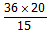= 48 days

2. A Canteen requires of 525 kg of wheat every week. How many kilograms of wheat is required in the month of Sept.

Solutions: Wheat required in 7 days = 525 kg

Wheat required in 1 day = 525 / 7

Wheat required in 30 days =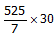=  2250 kg

3) The cost of 8 diaries and 12 calendars is Rs. 3138. Find the cost of 12 diaries and 18 calendars.

Solutions: 8 diaries and 12 calendars cost Rs. 3138

12 diaries and 18 calendars are 3/2 times of 8 diaries and 12 calendars. So the cost is also 3/2 times the cost of Rs. 3138.

So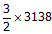= Rs. 4707

4. Total cost of 5 pens and 6 pencils is Rs. 84. The cost of pencil is equal to one third of the cost of a pen. Find the cost of 4 pens and 5 pencils.

Solutions: It is given that cost of one pencil = 1/3 the cost of a pen

So 6 pencils = 6/3 pens = 2 pens

Cost of 5 pens and 6 pencils = cost of 5 pens + 2 pens

So Cost of 7 pens = Rs. 84

So cost of one pen is 84/7 = Rs. 12

And cost of one pencil = 12/3 = Rs. 4

Cost of 4 pens and 5 pencils = 4 x 12 + 5 x 4 = Rs. 68

5. 300 apples are equally distributed amongst certain number of students. If there were 10 more students, each will get one apple less. Find total number of students.

Solution: Let the number of students be x

Each will get (300 / x) apples.

If no. of students is x + 10 each will get (300 / x) – 1 apples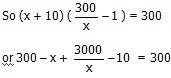or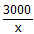– x = 10

or 3000 – x2 = 10x

or x2 + 10x – 3000 = 0

or x2 + 60x – 50x – 3000 = 0

or x (x + 60) – 50 (x + 60) = 0

(x – 50) (x + 60) = 0

X = 50 or - 60

So x = 50

6. One seventh of a number is 51. Find 64% of that number

Solutions: One seventh of a member is 51

So the number is 51 x 7 = 357

64% of 357 = 357 x (64 / 100) = 5712 / 25 = 228.48

7. A certain number of sweets were distributed among 56 children, equally. Each child got 8 sweets and 17 sweets were left. Find the total number of sweets?

Solutions: Total sweets = 56 x 8 + 17

= 448 + 17 = 465

8. A person takes 3 minutes to write a letter. During 10 am to 12 noon 1960 letters are to be written. How many persons should be employed to complete this job in time.

Solutions: No. of person to be employed =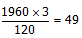9. Sweets were to be distributed equally among 200 children. 40 children were absent, so each child got 2 sweets extra. How many sweets were distributed?

Solution: Let the sweets be x

Each child were to get (x / 200) sweets

Children presence = 200 – 40 = 160

and each child got x / 200 + 2 sweets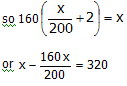or 40x / 200 = 320 or x =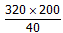= 1600 sweets

The document Unitary Method | Quantitative Techniques for CLAT is a part of the CLAT Course Quantitative Techniques for CLAT.
All you need of CLAT at this link: CLAT

## Quantitative Techniques for CLAT

46 videos|69 docs|95 tests

## FAQs on Unitary Method - Quantitative Techniques for CLAT

 1. What is the unitary method in mathematics?Ans. The unitary method is a mathematical technique used to solve problems involving proportional relationships. It helps in finding the value of an unknown quantity by using the concept of proportionality.
 2. How is the unitary method applied in real-life situations?Ans. The unitary method is commonly used in everyday life situations such as calculating prices of items, determining quantities for recipes, finding distances based on speed and time, and analyzing proportions in various scenarios.
 3. Can you provide an example of using the unitary method to solve a problem?Ans. Sure! Let's say a car travels 200 kilometers in 4 hours. To find the distance it can travel in 8 hours, we can use the unitary method. Since the time has doubled, we can assume the distance will also double. Therefore, the car can travel 400 kilometers in 8 hours.
 4. Are there any limitations to using the unitary method?Ans. While the unitary method is a useful tool, it may not be applicable in all situations. It assumes a direct proportionality between the given quantities, which may not always be the case in real-life scenarios. Additionally, it is important to consider any constraints or limitations specific to the problem at hand.
 5. How can I improve my skills in applying the unitary method?Ans. To improve your skills in using the unitary method, practice solving a variety of problems that involve proportions and ratios. Familiarize yourself with different types of unitary method questions and try to apply the concept to real-life situations. Additionally, seeking guidance from teachers or using online resources for practice can also enhance your understanding and proficiency in using the unitary method.

## Quantitative Techniques for CLAT

46 videos|69 docs|95 testsExplore Courses for CLAT examSignup to see your scores go up within 7 days! Learn & Practice with 1000+ FREE Notes, Videos & Tests.
10M+ students study on EduRev
Track your progress, build streaks, highlight & save important lessons and more!
Related Searches

,

,

,

,

,

,

,

,

,

,

,

,

,

,

,

,

,

,

,

,

,

;